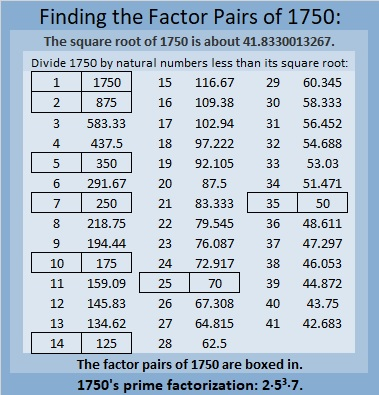# 1750 and Level 1

Contents

### Today’s Puzzle:

Place the numbers 1 to 12 in the first column and also in the top row so that those numbers are the factors of the given clues.### Factors of 1750:

• 1750 is a composite number.
• Prime factorization: 1750 = 2 × 5 × 5 × 5 × 7, which can be written 1750 = 2 × 5³ × 7.
• 1750 has at least one exponent greater than 1 in its prime factorization so √1750 can be simplified. Taking the factor pair from the factor pair table below with the largest square number factor, we get √1750 = (√25)(√70) = 5√70.
• The exponents in the prime factorization are 1,3 and 1. Adding one to each exponent and multiplying we get (1 + 1)(3 + 1)(1 + 1) = 2 × 4 × 2 = 16. Therefore 1750 has exactly 16 factors.
• The factors of 1750 are outlined with their factor pair partners in the graphic below.### More About the Number 1750:

1750 is the hypotenuse of THREE Pythagorean triples:
490 1680 1750, which is (7-24-25) times 70,
616 1638 1750, which is 14 times (44-117-125), and
1050 1400 1750, which is (3-4-5) times 350.

1750 looks interesting in some other bases:
It’s 5050 in base 7 because 5(7³) + 5(7) = 1750, and it’s
1K1 in base 33 because 1(33²) + 20(33) + 1(1) = 1750.

This site uses Akismet to reduce spam. Learn how your comment data is processed.